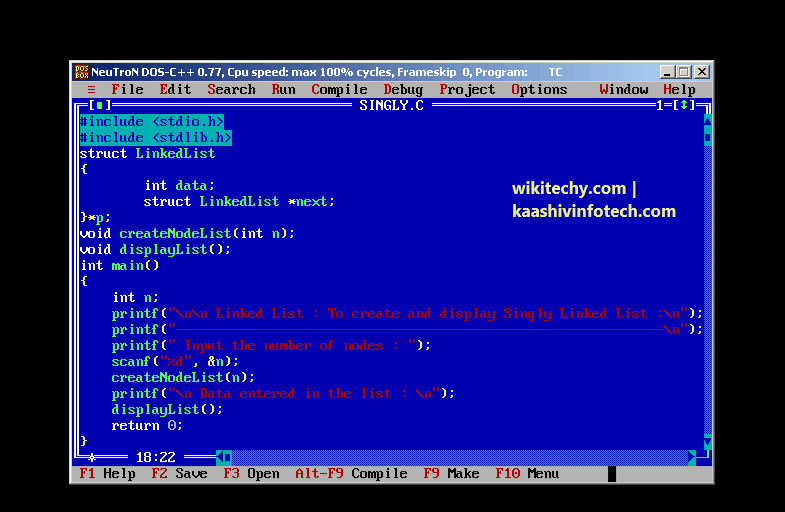## Structure and Linked List with C Programming in Tamil

• A linked list is formed when many such nodes are linked together to form a chain.
• Each node points to the next node present in the order.
• The first node is always used as a reference to traverse the list and is called HEAD. The last node points to NULL.## Node

• A node is a collection of two sub-elements or parts. A data part that stores the element and a next part that stores the link to the next node.Node

## Example

• The above definition is used to create every node in the list.
• The data field stores the element and the next is a pointer to store the address of the next node.
• In place of a data type, struct LinkedList is written before next.
• That's because its a self-referencing pointer. It means a pointer that points to whatever it is a part of.
• Here next is a part of a node and it will point to the next node.## Creating a Node

• typedef is used to define a data type in C.
• malloc() is used to dynamically allocate a single block of memory in C, it is available in the header file stdlib.h.
• sizeof() is used to determine size in bytes of an element in C. Here it is used to determine size of each node and sent as a parameter to malloc.
• The below code will create a node with data as value and next pointing to NULL.Creating a node

## Example

• Here the new node will always be added after the last node. This is known as inserting a node at the rear end.
• The last node is checked by the condition : ` p->next = NULL; `
• Here -> is used to access next sub element of node p. NULL denotes no node exists after the current node, i.e. its the end of the list.

## Example

• The linked list can be traversed in a while loop by using the head node as a starting reference: node p;Learn C - C tutorial - Linked List Code - C examples - C programs